# Dataframe groupby date and time

In this post we will see how to group a timeseries dataframe by Year,Month, Weeks or days. Additionally, we will also see how to groupby time objects like hours

We will use Pandas grouper class that allows an user to define a groupby instructions for an object

Along with grouper we will also use dataframe Resample function to groupby Date and Time. It is used for frequency conversion and resampling of time series

## Pandas Grouper

pandas.Grouper(key=None, level=None, freq=None, axis=0, sort=False)[source]

This specification will select a column via the key parameter, or if the level and/or axis parameters are given, a level of the index of the target object.

Let’s jump in to understand how grouper works

### Create a TimeSeries Dataframe

``````from datetime import datetime
from pandas import DataFrame
df\_original = DataFrame(
{
"Name": "Maria Maria Maria Maria Jane Carlos".split(),
"Sample": \[25, 9, 4, 3, 2, 8\],
"Date": \[
datetime(2019, 9, 1, 13, 0),
datetime(2019, 9, 1, 13, 5),
datetime(2019, 10, 1, 20, 0),
datetime(2019, 10, 3, 10, 0),
datetime(2019, 12, 2, 12, 0),
datetime(2019, 9, 2, 14, 0),
\],
}
)
df\_original.set\_index('Date',inplace=True)
``````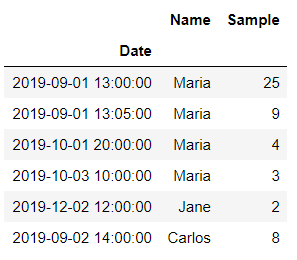### GroupBy Month

We are using pd.Grouper class to group the dataframe using key and freq column.

Parameter key is the Groupby key, which selects the grouping column and freq param is used to define the frequency only if  if the target selection (via key or level) is a datetime-like object

Freq can be Hourly, Daily, Weekly, Monthly etc. Full specification of available frequency can be found here

``````import pandas as pd
df_original.groupby(pd.Grouper(key='Date',freq='M')).sum()
``````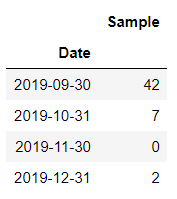### GroupBy 5 days

We will set the freq parameter as 5D here and key will be Date column. You can see the second, third row Sample value as 0.

Because we have used frequency of 5 days(5D) so if there is no data available for any dates in the original column then it returns 0

if the aggregate function is set to mean instead of sum then those 0’s will be replaced by NaN’s

``````import pandas as pd
df_original.groupby(pd.Grouper(key='Date',freq='5D')).sum()
``````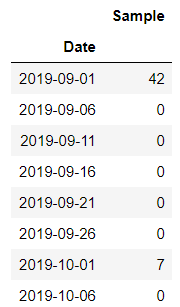Let’s filter out those 0 from the result and see only the Sample where a Non-Zero value exists

import pandas as pd df_original_5d = df_original.groupby(pd.Grouper(key=’Date’,freq=’5D’)).sum() df_original_5d[df_original_5d[‘Sample’]!=0]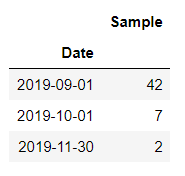### Groupby Level Parameter

Let’s set the index of the original dataframe to any of the target column we want to group

``````df_original.set_index('Name', inplace=True)
``````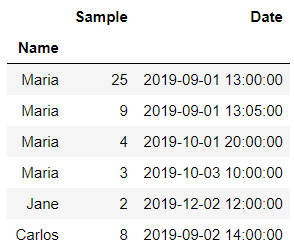Set the target column as dataframe index and then group by Index using the level parameter

All the Samples are summed up for each Name group

``````df_original.groupby(pd.Grouper(level='Name',axis=0)).sum()
``````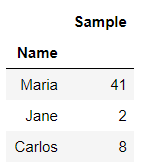You cannot use both Level and Key parameters together. It will throw an error with the following message: “The Grouper cannot specify both a key and a level!

### Groupby Year end Freq

Let’s create a dataframe with datetime index

``````df_original = DataFrame(
{
"Alpha": "A A A A A A A B".split(),
"Name": "Mana Pun Mana Mana Rosy Rosy Rosy Mana".split(),
"Sample": [10, 13, 5, 2, 9, 8, 5, 16],
"Date": [
datetime(2019, 1, 1, 13, 0),
datetime(2019, 1, 1, 13, 5),
datetime(2019, 10, 1, 20, 0),
datetime(2019, 10, 2, 10, 0),
datetime(2019, 10, 1, 20, 0),
datetime(2019, 10, 2, 10, 0),
datetime(2019, 12, 2, 12, 0),
datetime(2019, 12, 2, 14, 0),
],
}
).set_index("Date")
``````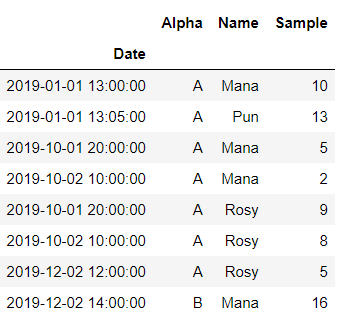We want to group this dataframe on Year End Frequency and it’s column Name

``````df_original.groupby([pd.Grouper(freq="A"), "Name"]).sum()
``````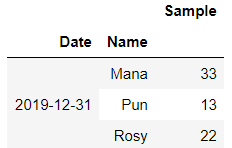## Groupby Date and Time using Resample

We will use resample function to group the timeseries. It is a convenience method for resampling and converting the frequency of any DatetimeIndex, PeriodIndex, or TimedeltaIndex

Let’s take our original dataframe and group it by Hour. We have to first set the Date column as Index

``````df_original.set_index('Date',inplace=True)
``````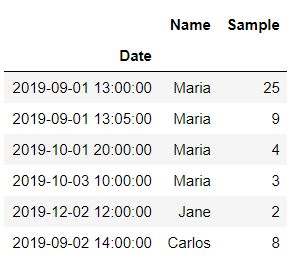Use resample function to group the dataframe by Hour. You can see NaN’s are included because in the original dataframe there are no values for those hours

``````df_original.resample('H').mean()
``````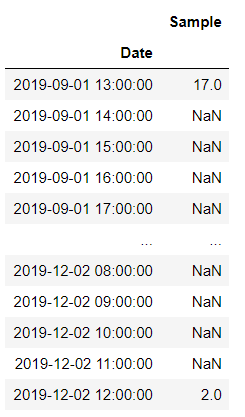Let’s group the original dataframe by Month using resample() function

``````df_original.resample('D').mean()
``````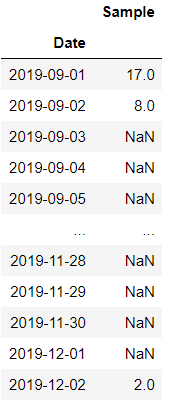We have used aggregate function mean to group the original dataframe daily. Days for which no values are available is set to NaN

## Conclusion

Here are the points to summarize that we have learnt so far about the Pandas grouper and resample functions

• Pandas Grouper class let user specify the groupby instructions for an object
• Select a column via the key parameter for grouping and provide the frequency to group with
• To use level parameter set the target column as the index and use axis to specify the axis along grouping to be done
• Groupby using frequency parameter can be done for various date and time object like Hourly, Daily, Weekly or Monthly
• Resample function is used to convert the frequency of DatetimeIndex, PeriodIndex, or TimedeltaIndex

Tags:

Categories:

Updated: